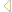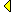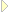# Time Conversion

### Kurt Nĝrmark ©    normark@cs.auc.dk    Department of Computer Science, Aalborg University, Denmark

 Abstract. This program transforms time from one representation to another. The source representation is the number of seconds elapsed since January 1, 1970, 00:00:00. The target representation is a vector of (year, month, day, hour, minute, second) all normalized in the sense introduced below.1.1  Time systems and functions
There are several different standards for representation of time on a Computer. Universal Time refers to a standard which counts the seconds since January 1, 1900 (midnight, GMT). In this paper we will use another fix point, namely January 1, 1970.

As an introductory observation, it is worthwhile to notice that the number of seconds since January 1, 1970 can is returned by the Scheme function current-time (taking no parameters) in some Scheme systems (SCM, for instance). The Scheme system gets this number from the underlying operating system, of course.

As an example, the current time was 948450887 at the moment of when these words were written. Furthermore

```  (time-decode 948450887) = (2000 1 21 10 34 47)
```
See details about time-decode in the next section. Please be aware of the problems of time zones and daylight saving time, cf. section 3.1

Scheme is weak with respect to static typing so let us here give the signature of the function:

```  time-decode: integer -> (year x month x day x hour x minute x second)
```
The type year represents an integer greater than or equal to 1970, month is the interval [1..12], day is [1..31], hour is [0..23], and both minute and second belong to [0..59]. The vector on the right hand side will be represented as a list in our program.

As a matter of terminology, the integer at the left hand side of the signature will be called the second counter. We say that an integer value is T-normalized if it is contained in the interval of type T. Thus, for instance, the integer 25 is not hour-normalized, but 1 is (leaving us with 24 hours which can be changed to one extra day).

In the one extreme we can imagine the conversions to be done via a (presumably complicated) formula which realizes an efficient calculation of the result, using arithmetic operators. In the other extreme we can imagine a simple solution based on successive counting and subtracting of well-defined time intervals such as years (with and without leap days), months (in four variations), days, and hours from the second counter. This would be a less efficient solution, but if reliability and trust are emphasized, this will provide for a transparent and easy to follow solution.

In the solution presented in section 2 we will allow "counting", but for some simple sub-problems we use solutions based on "calculation" with formulas involving simple arithmetic operators.2  The solutionIn this section we describe the solutions in a number of natural subsection. First we find the year. The last information we extract is the month.
 2.1  Dealing with years2.2  Dealing with days, hours, minutes, and seconds2.3  Dealing with months2.4  Putting it all together2.1  Dealing with years
The first subproblem is to find the year represented by the second counter. We will use the 'count and subtract' approach to find the year. Is is critical that we deal correctly with leap years. Therefore we start our programming with a function predicate
leap-year that determines whether a year is a leap year. The rule is that y is a leap year if it is divisible by 4, it is not a leap year if it is divisible by 100, but - as yet an exception - a leap year if it is divisible by 400. I do not know whether there are more exceptions, but we can be confident that these will not be relevant in our life time, nor in this program's life time. Notice, that the most extreme exception listed above is relevant in this year, 2000.

Now the function years-and-seconds solves the problem. The underlying counting is an iterative process, which we in Scheme handle by a tail-recursive function. This function is called cycle-years. The "cycling" starts from base-year. (cycle-years n y r) maintains the invariant

```  n + r = C
```
where C is the start value of r. n is increased and r is decreased by the the number of seconds in the actual year y. y is increased by one for each iteration. When the rest r becomes less than the seconds in the actual year, we return y and the rest seconds (as a list of two elements).

The function cycle-years depends on the constants seconds-in-a-leap-year and seconds-in-a-normal-year which are easily pre-calculated using a pocket calculator.2.2  Dealing with days, hours, minutes, and seconds
Now we have reduced the problem to finding the normalized months, days, hours, minutes and seconds from a rest second counter r that is less than the number of seconds in a year. It would be natural to find the month next, but doing so would call for yet another counting process, because a month is an irregular time interval (some months have 31 days, others 30, February has normally 28 days, but there is 29 days in leap years).2.3  Dealing with months
In section
2.2 we almost solved the rest of the problems. However, we still have to find the month component from a non day-normalized number of days. As an example, we may have 45 days, which should represent February 14. As another example, day counter 60 represents February 29 in a leap year, and March 1 in non-leap years. Thus, we clearly see that we need to take the actual year into account when finding the normalized months and days from a day counter.

The function day-and-month solves the problem. Like in section 2.1 we go for a counting solution. This is done by the tail-recursive helping function day-and-month-help. A call of

``` (day-and-month-help n m y c)
```
involves that in year y and month m we are taking
```  (days-in-month m y)
```
days from c and adding a month to m. The function days-in-month relies on a table of month lengths (for a normal year) called month-length-normal-year. The iteration goes on as long as the rest days, c, is less than the number of days in the actual month m (in the year y).

In the function day-and-month we pass the expression (+ 1 day-count) to the formal day counter parameter c in day-and-month-help. The reason is that one day into January brings us to some point in time at January 2, not January 1. Thus, because c will be the day of the month, c can never be 0. In the tail-recursive call, we know that c > (days-in-month m y), thus ensuring this property recursively.3  Post ScriptumIn this section we will make a final remark to the time conversion program.
 3.1  Final remarks3.1  Final remarks
As a final remark it may be noticed that time zones and daylight saving time may cause problems for the time conversion. Being in a specific time zone, or going from normal "winter time" to daylight saving time does probably not change the second counter in the computer. Consequently, we need to take corrective actions in the program in order to return the right time from the conversion function .

The easiest counter measure is to add or subtract an hour (3600 seconds) in time-decode just after the parameter n (the second counter) has been passed as parameter.

This concludes the time conversion example.

It would be possible - and natural - to continue the example with the reverse transformation, by calculation of the weekday, and by transformation of time intervals. Most of these can be found in the LAML library called Time, cf. the LAML libraries